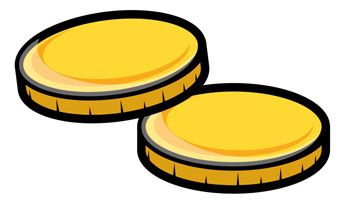What are the odds that Dr. Johnson tosses two coins and get one “head” and one “tail”?

See the answer

Answer:

There are 4 possible outcomes for this event: HH, HT, TT, TH (H stands for the “heads” and T stands for the “tails”). There are two tosses with one “head” and one “tail”: HT and TH. So the probability is 2/4 =1/2. Therefore, the odds are 50%.

Facebook Comments